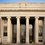# Discussion: Rotated Capacitor Plate

Consider a charged capacitor made with two square plates of side length $$L$$, uniformly charged, and separated by a very small distance $$d$$. The EMF across the capacitor is $$\xi$$. One of the plates is now rotated by a very small angle $$\theta$$ to the original axis of the capacitor. Find an expression for the difference in charge between the two plates of the capacitor, in terms of (if necessary) $$d$$, $$\theta$$, $$\xi$$, and $$L$$.

Also, approximate your expression by transforming it to algebraic form: i.e. without any non-algebraic functions. For example, logarithms and trigonometric functions are considered non-algebraic. Assume $d << L$ and $\theta \approx 0$.

Hint: You may assume that $\frac {\theta L}{d}$ is also very small.

Note: This problem was originally proposed by Trung Phan for the IPhOO.Note by Ahaan Rungta
7 years, 3 months ago

This discussion board is a place to discuss our Daily Challenges and the math and science related to those challenges. Explanations are more than just a solution — they should explain the steps and thinking strategies that you used to obtain the solution. Comments should further the discussion of math and science.

When posting on Brilliant:

• Use the emojis to react to an explanation, whether you're congratulating a job well done , or just really confused .
• Ask specific questions about the challenge or the steps in somebody's explanation. Well-posed questions can add a lot to the discussion, but posting "I don't understand!" doesn't help anyone.
• Try to contribute something new to the discussion, whether it is an extension, generalization or other idea related to the challenge.

MarkdownAppears as
*italics* or _italics_ italics
**bold** or __bold__ bold
- bulleted- list
• bulleted
• list
1. numbered2. list
1. numbered
2. list
Note: you must add a full line of space before and after lists for them to show up correctly
paragraph 1paragraph 2

paragraph 1

paragraph 2

[example link](https://brilliant.org)example link
> This is a quote
This is a quote
    # I indented these lines
# 4 spaces, and now they show
# up as a code block.

print "hello world"
# I indented these lines
# 4 spaces, and now they show
# up as a code block.

print "hello world"
MathAppears as
Remember to wrap math in $$ ... $$ or $ ... $ to ensure proper formatting.
2 \times 3 $2 \times 3$
2^{34} $2^{34}$
a_{i-1} $a_{i-1}$
\frac{2}{3} $\frac{2}{3}$
\sqrt{2} $\sqrt{2}$
\sum_{i=1}^3 $\sum_{i=1}^3$
\sin \theta $\sin \theta$
\boxed{123} $\boxed{123}$

Sort by:

Draw two rotated squares and find area common to both. Find the new capacitance, and apply $Q = C \zeta$, to get $\triangle Q = Q - (-Q ) = 2C \zeta$

It is easy to find the overlapping area as $\displaystyle A = L^2 \bigg(1 - \frac{2 \tan \theta}{(1+ \tan \theta + \sec \theta)^2}\bigg)$

Hence, $\displaystyle C = \frac{\epsilon_{0} A }{d} = \frac{\epsilon L^2}{d} \bigg(1 - \frac{2 \tan \theta}{(1+ \tan \theta + \sec \theta)^2}\bigg)$

Thus, $\displaystyle \triangle Q = \frac{2 \epsilon_{0} L^2 \zeta }{d} \bigg(1 - \frac{2 \tan \theta}{(1+ \tan \theta + \sec \theta)^2}\bigg)$

When $\displaystyle \theta \approx 0$, $\triangle Q \approx \frac{2 \epsilon_{0} L^2 \zeta }{d} \bigg(1 - \frac{\theta}{2}\bigg)$

- 7 years, 3 months ago